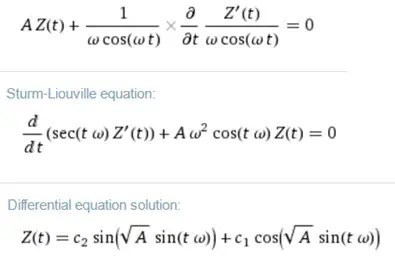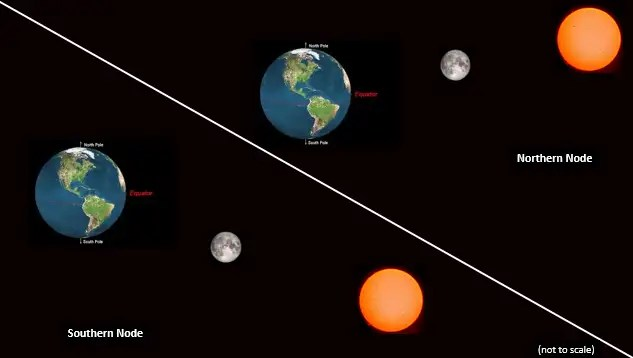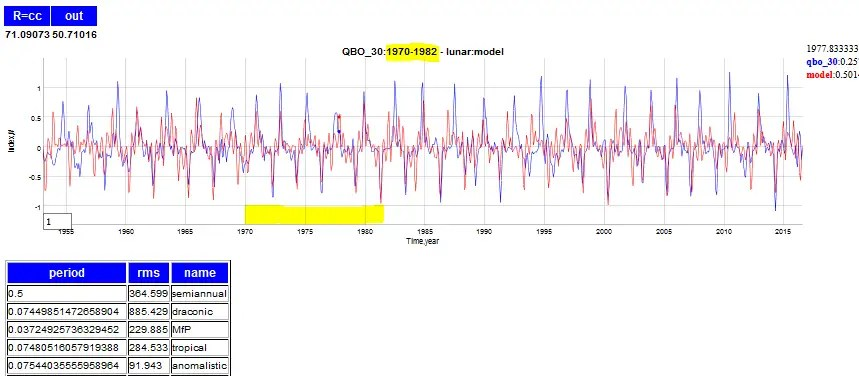# Compact QBO Derivation

[mathjax]
This is a concise derivation for a model of QBO by reducing Laplace’s tidal equations along the equator.

## Part 1 : Deriving a closed-form solution

For a fluid sheet of average thickness $$D$$, the vertical tidal elevation $$zeta$$, as well as the horizontal velocity components $$u$$ and $$v$$ (in the latitude $$varphi$$ and longitude $$lambda$$ directions).

This is the set of Laplace’s tidal equations (wikipedia). Along the equator, for $$varphi$$ at zero we can reduce this.{begin{aligned}{frac {partial zeta }{partial t}}+{frac {1}{acos(varphi )}}left[{frac {partial }{partial lambda }}(uD)+{frac {partial }{partial varphi }}left(vDcos(varphi )right)right]=0,\{frac {partial u}{partial t}}-vleft(2Omega sin(varphi )right)+{frac {1}{acos(varphi )}}{frac {partial }{partial lambda }}left(gzeta +Uright)=0,\{frac {partial v}{partial t}}+uleft(2Omega sin(varphi )right)+{frac {1}{a}}{frac {partial }{partial varphi }}left(gzeta +Uright)=0,end{aligned}}

where $$Omega$$ is the angular frequency of the planet’s rotation, $$g$$ is the planet’s gravitational acceleration at the mean ocean surface, $$a$$ is the planetary radius, and $$U$$ is the external gravitational tidal-forcing potential.

The main candidates for removal due to the small-angle approximation along the equator are the second terms in the second and third equation. The plan is to then substitute the isolated $$u$$ and $$v$$ terms into the first equation, after taking another derivative of that equation with respect to $$t$$.{begin{aligned}{frac {partial zeta }{partial t}}+{frac {1}{a}}left[{frac {partial }{partial lambda }}(uD)+{frac {partial }{partial varphi }}left(vDright)right]=0,\{frac {partial u}{partial t}}+{frac {1}{a}}{frac {partial }{partial lambda }}left(gzeta +Uright)=0,\{frac {partial v}{partial t}}+{frac {1}{a}}{frac {partial }{partial varphi }}left(gzeta +Uright)=0,end{aligned}}

Taking another derivative of the first equation:{begin{aligned}{afrac {partial^2 zeta }{partial t^2}}&+ frac {partial }{partial t} left[{frac {partial }{partial lambda }}(uD)+{frac {partial }{partial varphi }}left(vDright)right]=0,end{aligned}}

Next, on the bracketed pair we invert the order of derivatives (and pull out the constant $$D$$){begin{aligned}{afrac {partial^2 zeta }{partial t^2}}&+ D left[{frac {partial }{partial lambda }}( frac {partial u }{partial t} )+{frac {partial }{partial varphi }}(frac {partial v }{partial t} )right]=0,end{aligned}}

Notice now that the bracketed terms can be replaced by the 2nd and 3rd of Laplace’s equations{begin{aligned}{frac {partial u}{partial t}}&=-{frac {1}{a}}{frac {partial }{partial lambda }}left(gzeta +Uright)end{aligned}}{begin{aligned}{frac {partial v}{partial t}}&=-{frac {1}{a}}{frac {partial }{partial varphi }}left(gzeta +Uright)end{aligned}}

as{begin{aligned}{a^2frac {partial^2 zeta }{partial t^2}}&- D left[{frac {partial }{partial lambda }}( {{frac {partial }{partial lambda }}left(gzeta +Uright)} )+{frac {partial }{partial varphi }}({{frac {partial }{partial varphi }}left(gzeta +Uright)})right]=0end{aligned}}

The $$lambda$$ terms are in longitude so that we can use a separation of variables approach and create a spatial standing wave for QBO, $$SW(s)$$ where $$s$$ is a wavenumber.{begin{aligned}{frac {partial^2 zeta }{partial t^2}}&- D left[( SW(s) zeta )+{frac {partial }{partial varphi }}({{frac {partial }{partial varphi }}left(gzeta +Uright)})right]=0end{aligned}}That is essentially close to a variation of a Sturm-Liouville equation (see right). To solve this for a plausible set of boundary conditions, we make a  connection between a change in latitudinal forcing with a temporal change:begin{aligned} {frac {partial zeta }{partial varphi } = frac {partial zeta }{partial t} frac {partial t }{partial varphi } } end{aligned}

After properly applying the chain rule, this reduces the equation to a function of $$zeta(t)$$ and $$varphi(t)$$, along with a constant A.$A zeta(t) + frac {1}{ frac {partial varphi }{partial t }} cdot frac {partial}{partial t}frac {zeta'(t)}{ frac {partial varphi }{partial t }} = 0$

So if we fix $$varphi(t)$$ to a periodic function with a long-term mean of zerobegin{aligned} frac {partial varphi }{partial t } = sum_{i=1}^{i=N} k_i omega_i cos(omega_i t) end{aligned}

to describe the tractive latitudinal displacement terms near the equator, then the solution is the following:begin{aligned} zeta(t) = sin( sqrt{A} sum_{i=1}^{i=N} k_i sin(omega_i t) + theta_0 ) end{aligned}

where $$A$$ is an aggregate of the constants of the differential equation and $$theta_0$$ represents the fixed phase offset necessary for aligning on a seasonal peak. This approximation of a tangential tractive force as a cyclic displacement for $$varphi(t)$$ is a subtle yet very effective means of eliminating a big unknown in the dynamics ( this is essentially similar to a Berry phase applied as a cyclic adiabatic process, with the phase being the internal sin modulation ). Not including this approximation leaves us with an indeterminate set of equations.

Now consider that the QBO itself is precisely the $$v(t)$$ term – the horizontal longitudinal velocity of the fluid, i.e. the wind in other words – which can be derived from the above by applying the solution to Laplace’s third tidal equation in simplified form above:$frac {partial v}{partial t} = cos( sqrt{A} sum_{i=1}^{i=N} k_i sin(omega_i t) + theta_0 )$

So the acceleration of wind, not the velocity, is what obeys the Sturm-Liouville equation. The acceleration of wind is simply a F=ma response to a tractive gravitational forcing. A derivative preserves the periods of the Fourier components, but not the amplitudes, so what we see is a differently shaped envelope for QBO — i.e. one that is more spiky due to the time derivative applied.

## Part 2 : Deriving the lunar forcing periods

We apply a seasonal aliasing of the lunar gravitational pull to generate the latitudinal tractive forcing terms above.  This turns into a set of harmonics which we derive next.

The starting premise is that a known lunar tidal forcing signal is periodic$L(t) = k cdot sin(omega_L t + phi)$

The seasonal signal is likely a strong periodic delta function, which peaks at a specific time of the year. This can be approximated as a Fourier series of period $$2pi$$.$s(t) = sumlimits_{i=1}^n a_i sin(2 pi t i +theta_i)$

For now, the exact form of this doesn’t matter, as what we are trying to show is how the aliasing comes about.

The forcing is then a combination of the lunar cycles $$L(t)$$ amplified in some way by the strongly cyclically peaked seasonal signal $$s(t)$$.$f(t) = s(t) L(t)$

Multiplying this out, and pulling the lunar factor into the sum$f(t) = k sumlimits_{i=1}^n a_i sin(omega_L t + phi) sin(2 pi t i +theta_i)$

then with the trig identity$sin(x) sin(y) = frac{1}{2} (cos(x-y)-cos(x+y))$

Expanding the lower frequency difference terms and ignoring the higher frequency additive terms$f(t) = k/2 sumlimits_{i=1}^n a_i sin((omega_L - 2 pi i)t +psi_i) + ...$

Thus we can now understand how the high frequency lunar tidal $$omega_L$$ terms get reduced in frequency by multiples of $$2pi$$, until it nears the period of the seasonal cycle. These are the Fourier harmonics of the aliased lunar cycles that describe the tractive forcing.

## Application

The Part 1 derivation provides the closed-form natural response and Part 2 provides the boundary condition forcing terms due to the lunar nodal cycle.To create a multiple linear regression fit, we consider all the aliased Fourier factors that are known from the lunisolar tidal cycles. What the regression does is determine the weighting of the $$a_i$$ terms, across the set of lunar $$omega_L$$ terms.In the regression fit shown above, the training region is 1970 to 1982, and the rest is extrapolated. To get back the wind speed QBO, the curves are integrated:Again the model fitting is only conducted on the interval from 1970 to 1982. Outside of that interval the model doesn’t track every peak but enough of the fine detail is captured that it is quite obvious that the model has predictive power.

## Conclusions

This acceleration version of the QBO is spikier than the more smoothly oscillating conventional wind speed description of QBO. Yet this spikiness makes sense as it is physically representative of the seasonally modulated lunar forcing. A straightforward time integration of the acceleration model will recover the wind speed version of the QBO.

Besides the excursion-limiting behavior imposed by a $$sin(sin(t))$$ modulation, this formulation can also show amplitude folding at the positive and negative extremes. In other words, if the amplitude is too large, the outer $$sin$$ modulation starts to shrink the excursion, instead of just limiting it. (edit 6/22/2017: When the amplitude starts folding and bifurcating in this fashion, it falls into the behavioral category identified as atmospheric wave breaking.)

By carefully applying these factors within the tidal formulation, we can generate a concise solution that has all the characteristics of the measured QBO. This needs to be adopted as the de facto QBO model which replaces the original formulation proposed by Lindzen in the late 1960’s .

 R. S. Lindzen and J. R. Holton, “A theory of the quasi-biennial oscillation,” Journal of the Atmospheric Sciences, vol. 25, no. 6, pp. 1095–1107, 1968.